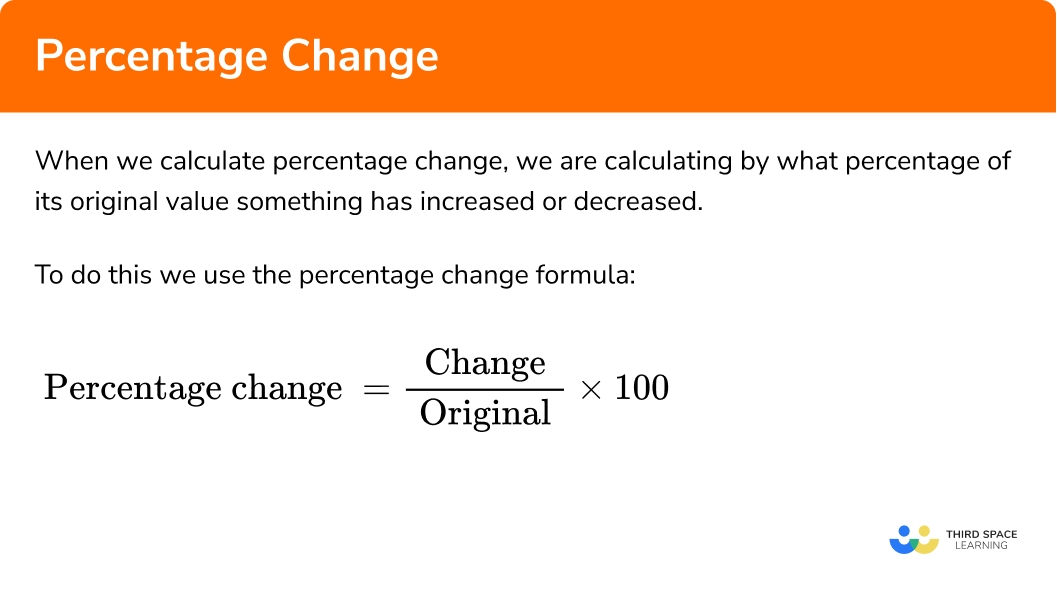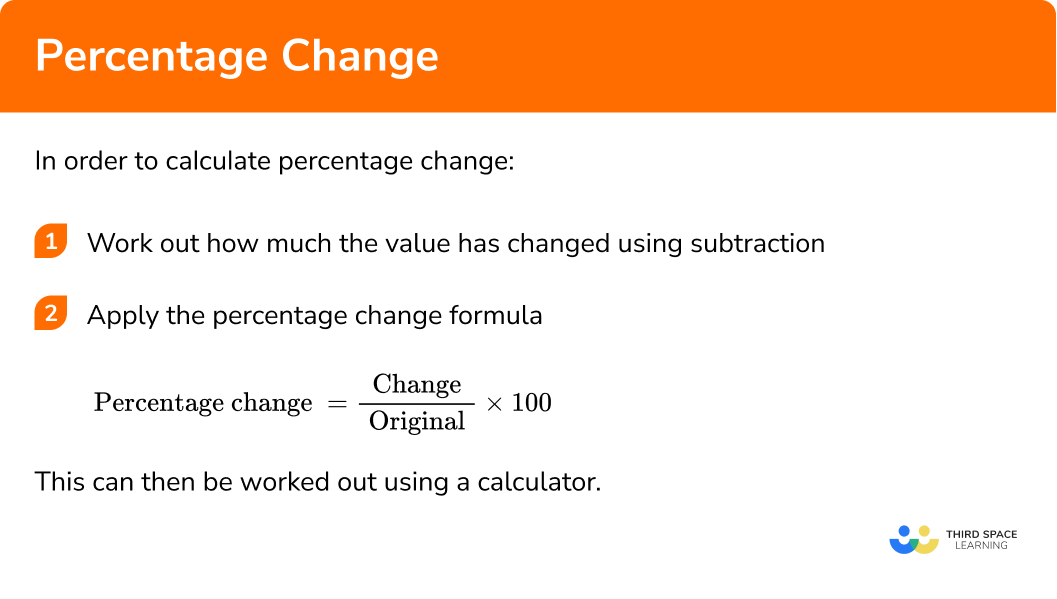GCSE Maths Number FDP Percentages

Percentage Change

# Percentage Change

Here is everything you need to know about calculating percentage change for GCSE maths (Edexcel, AQA and OCR). You’ll learn how to calculate the percentage by which a value has increased or decreased.

Look out for the percentage change worksheets and exam questions at the end.

## What is percentage change?

When we calculate percentage change, we are calculating by what percentage of its original value something has changed.

To do this we use the percentage change formula:

$\text { Percentage change }=\frac{\text { Change }}{\text { Original }} \times 100$

Percentage change can also be called percentage increase, percentage decrease, percentage profit, percentage gain, percentage reduction or percentage loss.

### What is percentage change?## How to calculate percentage change

In order to calculate percentage change:

1. Work out how much the value has changed using subtraction.
2. Apply the percentage change formula.
$\text { Percentage change }=\frac{\text { Change }}{\text { Original }} \times 100$

This can then be worked out using a calculator.

### Explain how to calculate percentage change in 2 steps## Percentage changeexamples

### Example 1: using a calculator

The weight of a baby has increased from 4.4kg to 5.06kg Find the percentage difference in the baby’s weight.

1. The baby’s weight has increased from 4.4kg to 5.06kg. Calculate the change.

$5.06 – 4.4 = 0.66kg$

2 The change is 0.66 and the original number is 4.4. Apply the percentage change formula.

\begin{aligned} \text { Percentage change }&=\frac{\text { Change }}{\text { Original }} \times 100 \\\\ \text { Percentage change }&=\frac{0.66}{4.4} \times 100 \\\\ \text { Percentage change }&=15 \% \end{aligned}

### Example 2: using a calculator

Anna bought a car for £6500. 2 years later Anna sold the car for £4160. Calculate Anna’s percentage loss.

£6500 – £4160 = £2340

\begin{aligned} \text { Percentage change }&=\frac{\text { Change }}{\text { Original }} \times 100 \\\\ \text { Percentage change }&=\frac{2340}{6500} \times 100 \\\\ \text { Percentage change }&=36 \% \end{aligned}

## How to calculate percentage change without a calculator

The initial steps are the same as when using a calculator. If you are not using a calculator, you will need to use your knowledge of fractions and decimals to find the percentage change.

1 Work out how much the value has changed using subtraction.

2 Apply the percentage change formula.

$\text { Percentage change }=\frac{\text { Change }}{\text { Original }} \times 100$

3 Simplify the fraction if necessary using equivalent fractions.

4 Convert the fraction to a decimal.

5 Calculate percentage change.

### Example 3: non-calculator

Matthew bought an antique for £360. He sold it for £396. Calculate Mattew’s percentage profit.

£396 – £360 = £36

\begin{aligned} \text { Percentage change }&=\frac{\text { Change }}{\text { Original }} \times 100 \\\\ \text { Percentage change }&=\frac{36}{360} \times 100 \ \end{aligned}

\begin{aligned} &\frac{36}{360} \text { can be simplified to } \frac{1}{10}\\\\ &\text { Percentage change }=\frac{1}{10} \times 100 \end{aligned}

$\begin{array}{l} \frac{1}{10}=0.1 \\\\ \text { Percentage change }=0.1 \times 100 \end{array}$

$\begin{array}{l} 0.1 \times 100=10 \\\\ \text { Percentage change }=10 \% \end{array}$

### Example 4: non-calculator

A clothes shop reduced the price of a jacket from £48 to £36. Find the percentage reduction.

£48 – £36 = £12

\begin{aligned} \text { Percentage change }&=\frac{\text { Change }}{\text { Original }} \times 100 \\\\ \text { Percentage change }&=\frac{12}{48} \times 100 \ \end{aligned}

\begin{aligned} &\frac{12}{48} \text { can be simplified to } \frac{1}{4}\\\\ &\text { Percentage change }=\frac{1}{4} \times 100 \end{aligned}

$\begin{array}{l} \frac{1}{4}=0.25 \\\\ \text { Percentage change }=0.25 \times 100 \end{array}$

$\begin{array}{l} 0.25 \times 100=25 \\\\ \text { Percentage change }=25 \% \end{array}$

## How to calculate more than one percentage change

Sometimes, a product in a shop may be discounted twice or the value of something may increase and then decrease. It is common in this case to think that we can just add the percentage changes together. But this does not work.

For example, if something is reduced by 10% in a sale and then the sale price is reduced by 10%, this does not mean a total reduction of 20%. This is because 10% of the sale price is not the same as 10% of the original price.

To calculate the total percentage change after two percentage changes:

1. Apply the first percentage change.
2. Apply the second percentage change.
3. Calculate the percentage change between the final value and the original value.

### Example 5: double percentage reduction

A television costing £500 was reduced by 10% in a sale. 2 weeks later the sale price was reduced by 10%. Find the overall percentage reduction in price.

10% of £500 = £50

£500 − £50 = £450

10% of £450 = £45

£450 − £45 = £405

The old value is £500 and the new value is £405.

Change: £500 − £405 = £95

\begin{aligned} \text { Percentage change }&=\frac{95}{500} \times 100 \\\\ \text { Percentage change }&=19 \% \end{aligned}

### Example 6: percentage decrease and increase

Holly bought a house for £240000 in 2015. By 2017 the value of the house had decreased by 5%. By 2020 the value of the house had increased by 20% from its value in 2017.

Find the overall percentage change in the value of the house.

5% of £240000 = £12000

£240000 − £12000 = £228000

20% of £228000 = £45600

£228000 + £45600 = £273600

Change: £273600 − £240000 = £33600

\begin{aligned} \text { Percentage change }&=\frac{33600}{240000} \times 100 \\\\ \text { Percentage change }&=14 \% \text { increase } \end{aligned}

### Common misconceptions

• Using an incorrect value for the denominator in the percentage increase formula

Using the new value instead of the original value for the denominator when calculating percentage change.

• Multiple percentage changes

Adding the percentages together to find the total percentage change. Remember that a percentage of an already increased or decreased value will be different to that percentage of the original value.

Percentage change is part of our series of lessons to support revision on percentages. You may find it helpful to start with the main percentages lesson for a summary of what to expect, or use the step by step guides below for further detail on individual topics. Other lessons in this series include:

### Practice percentage change questions

Calculate the percentage change that has occurred in each of these questions.

You may use a calculator for questions 1, 2, 5 and 6.

1. \pounds 400 increased to \pounds 568 .

168\%42\%68\%48\%The change is \pounds 168 , so the percentage change is \frac{168}{400} \times 100 = 42\% .

2. 70kg reduced to 59.5kg .

16\%10.5\%59.5\%15\%The change is \pounds 10.50 , so the percentage change is \frac{10.5}{70} \times 100 = 15\% .

3. 60cm increased to 75cm

27.5\%12.5\%25\%15\%The change is 15cm , so the percentage change is \frac{15}{60} \times 100 = 25\% .

4. \pounds 90 reduced to \pounds 63

30\%70\%27\%63\%The change is \pounds 27 , so the percentage change is \frac{27}{90} \times 100 = 30\% .

5. 500l increased by 20\% and then increased again by a further 10\% .

30\%32\%20\%10\%The first change is 20\% , and the second percentage change is 10\% , which means the final amount is 660l .

The overall change is 160l , so the overall percentage change is \frac{160}{500} \times 100 = 32\% .

6. \pounds 6000 increased by 20\% and then decreased by 25\%

22.5\% decrease22.5\% increase10\% decrease10\% increaseThe first change is a 20\% increase so there is \pounds 7200 , and the second percentage change is a 25\% decrease, which means the final amount is \pounds 5400 .

The overall change is \pounds 600 , so the overall percentage change is \frac{600}{6000} \times 100 = 10\% .

### Percentage changeGCSE questions

Calculator

1. The number of people attending a hockey club has increased from 18 to 24 .

1 decimal place.

(3 marks)

Change: 24 − 18 = 6

(1)

\begin{aligned} \text { Percentage change }&=\frac{6}{18} \times 100 \\\\ \text { Percentage change }&=33.333… \% \end{aligned}

(1)

Percentage change = 33.3\%

(1)

2. (a) The number of bee-keepers in England increased from 15000 in 2008 to 29000 in 2013 .

Calculate the percentage increase.

(b) Since 2013 , the number of registered bee-keepers has increased by 52\% .

Find the total percentage increase in the number of bee-keepers between 2008 and now.

(7 marks)

(a)

Change: 29000  − 15000 = 14000

(1)

\begin{aligned} \text { Percentage change }&=\frac{14000}{15000} \times 100 \\\\ \text { Percentage change }&=93.3 \% \end{aligned}

(1)

(b)

52\% of 29000 = 15080

(1)

29000 + 15080 = 44080

(1)

Change: 44080  − 15000 = 29080

(1)

\begin{aligned} \text { Percentage change }&=\frac{29080}{15000} \times 100 \\\\ \text { Percentage change }&=193.8 \% \end{aligned}

(2)

Non-calculator

3. The number of hot drinks sold by a cafe decreased from 3500 in May to 3150 in June.

Calculate the percentage decrease.

(3 marks)

Change: 3500  − 3150 = 350

(1)

\begin{aligned} \text { Percentage change }&=\frac{350}{3500} \times 100 \\\\ \text { Percentage change }&=\frac{1}{10} \times 100 \\\\ \text { Percentage change }&=10 \% \end{aligned}

(2)

## Learning checklist

You have now learned how to:

• Calculate the percentage change between two values with and without a calculator
• Calculate the overall percent difference following multiple percent changes

## Still stuck?

Prepare your KS4 students for maths GCSEs success with Third Space Learning. Weekly online one to one GCSE maths revision lessons delivered by expert maths tutors.

Find out more about our GCSE maths tuition programme.